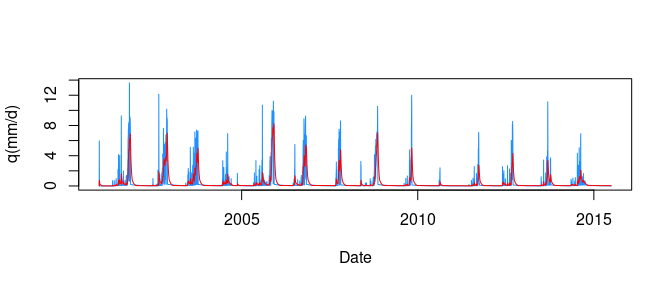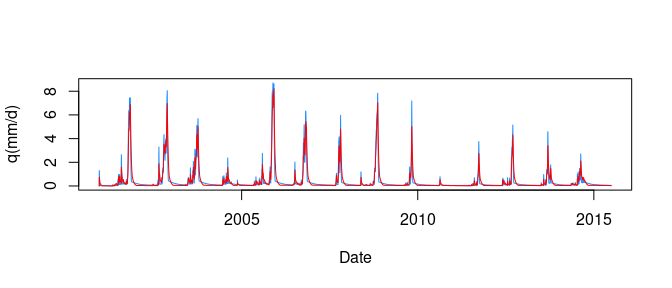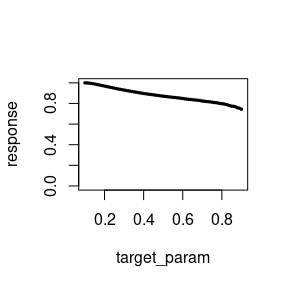# Chapter 2: A lumped model

## The problem

The following example is what I consider the first step to understand how HBV.IANIGLA works. As a first approach, I propose you to fit the streamflow discharge in a synthetic lumped catchment case (in my opinion this the most simple hydrological modeling case). The objectives of this excersice are:

• to introduce the building-up of the model.
• to get a first approach to parameter calibration.
• to fit the streamflow discharge in a perfect match case (this does not occur in real world hydrology).
• to make a sensitivity analysis.

## Data

Initially, we load the dataset containing all the necessary information to run the model: air temperature, precipitation, potential evapotranspiration and the outflow of our synthetic catchment:

library(HBV.IANIGLA)

data("lumped_hbv")

#>         Date T(ºC) P(mm/d) PET(mm/d) qout(mm/d)
#> 1 2000-12-16 12.72       0      0.00       0.00
#> 2 2000-12-17 15.23       0     20.00       0.63
#> 3 2000-12-18 15.31       0     19.99       0.74
#> 4 2000-12-19 12.82       0     19.99       0.63
#> 5 2000-12-20 10.45       0     19.98       0.53
#> 6 2000-12-21 11.05       0     19.96       0.43
summary(lumped_hbv)
#>       Date                T(ºC)             P(mm/d)         PET(mm/d)
#>  Min.   :2000-12-16   Min.   :-17.0300   Min.   : 0.000   Min.   : 0.000
#>  1st Qu.:2004-08-04   1st Qu.:  0.0025   1st Qu.: 0.000   1st Qu.: 2.960
#>  Median :2008-03-23   Median :  5.8550   Median : 0.000   Median : 9.910
#>  Mean   :2008-03-23   Mean   :  5.0681   Mean   : 1.183   Mean   : 9.992
#>  3rd Qu.:2011-11-10   3rd Qu.: 10.6375   3rd Qu.: 0.000   3rd Qu.:17.040
#>  Max.   :2015-06-30   Max.   : 17.6600   Max.   :97.800   Max.   :20.000
#>    qout(mm/d)
#>  Min.   :0.000
#>  1st Qu.:0.040
#>  Median :0.050
#>  Mean   :0.409
#>  3rd Qu.:0.250
#>  Max.   :8.230

## Building the model

This case is about a catchment without glaciers. In the next lines I will help you to build-up the model line by line. Because is our first excersice, I will provide you with the correct initial conditions and parameters in all modules except for the Routing_HBV function.

Remember that hydrological models are generally build in a top down direction (from precipitation to streamflow routing - note that most hydrological books are structured in the same way).

# we first consider the SnowGlacier module to take into account
# precipitation partioning and the snow accumulation/melting.
snow_module <-
SnowGlacier_HBV(model = 1,
inputData = as.matrix( lumped_hbv[ , c('T(ºC)', 'P(mm/d)')] ),
initCond = c(20, 2),
param = c(1.20, 1.00, 0.00, 2.5) )

# is always advisable to take a look at the output (unless until you get familiarised
# with the model)
#>      Prain Psnow SWE Msnow Total
#> [1,]     0     0   0    20    20
#> [2,]     0     0   0     0     0
#> [3,]     0     0   0     0     0
#> [4,]     0     0   0     0     0
#> [5,]     0     0   0     0     0
#> [6,]     0     0   0     0     0

# now see the function documentation...you should be able to relate the model option
# with the matrix output!

Now we will pass the total water produced (rainfall plus snowmelt) to the soil routine. Note that we will use the potential evapotranspiration series,

soil_module <-
Soil_HBV(model = 1,
inputData = cbind(snow_module[ , "Total"], lumped_hbv$PET(mm/d)), initCond = c(100, 1), param = c(200, 0.8, 1.15) ) head(soil_module) #> Rech Eac SM #> [1,] 9.012505 0.000000 110.98750 #> [2,] 0.000000 13.873437 97.11406 #> [3,] 0.000000 12.133188 84.98087 #> [4,] 0.000000 10.617298 74.36357 #> [5,] 0.000000 9.286151 65.07742 #> [6,] 0.000000 8.118408 56.95901 from this module we can get the actual evapotranspiration, soil moisture and recharge series. In the next step we incorporate the last variable to the routing function. Remember that the module’s parameters (param argument) are not calibrated! routing_module <- Routing_HBV(model = 1, lake = F, inputData = as.matrix(soil_module[ , "Rech"]), initCond = c(0, 0, 0), param = c(0.9, 0.01, 0.001, 0.5, 0.01) ) head(routing_module) #> Qg Q0 Q1 Q2 STZ SUZ #> [1,] 0.000000000 0.0000000 0.000000000 0.000000e+00 9.01250463 0.0000000 #> [2,] 7.661254163 7.6612542 0.000000000 0.000000e+00 0.85125046 0.5000000 #> [3,] 0.326035416 0.3161254 0.009900000 1.000000e-05 0.03512505 0.9801000 #> [4,] 0.010072240 0.0000000 0.010052250 1.999000e-05 0.00000000 0.9951728 #> [5,] 0.009881698 0.0000000 0.009851728 2.997001e-05 0.00000000 0.9753211 #> [6,] 0.009693151 0.0000000 0.009653211 3.994004e-05 0.00000000 0.9556679 #> SLZ #> [1,] 0.00000000 #> [2,] 0.00000000 #> [3,] 0.00999000 #> [4,] 0.01997001 #> [5,] 0.02994004 #> [6,] 0.03990010 Finally we will apply the transfer function in order to adjust the hydrograph timing, tf_module <- round( UH(model = 1, Qg = routing_module[ , "Qg"], param = c(1.5) ), 2) # let's plot the "true" and simulated hydrographs plot( x = lumped_hbv[ , "Date"], y = tf_module, type = "l", col = "dodgerblue", xlab = "Date", ylab = "q(mm/d)") lines(x = lumped_hbv[ , "Date"], y = lumped_hbv[ , "qout(mm/d)"], col = "red")# you can get a goodness of fit measurement by, cor(x = lumped_hbv[ , "qout(mm/d)"], y = tf_module) #>  0.7486402 # hint: to use hydrological goodness of fit functions (e.g.: NSE - Nash-Sutcliffe # Efficiency) you can install the hydroGOF package. As you will note, our HBV model needs some calibration (in he Routing_HBV parameters). Now is your turn change (manually) the corresponding parameter values to get a decent streamflow simulation. Help: the order of magnitude of the actual parameters is correct (e.g.: the real value of K0 is between 0.9 and 0.1 and so on). Hints When you are changing the parameters ‘by hand’ is always advisable to plot the results. Pay attention on how sensible is the result to changes in a single parameter. ## The HBV model as a function In my view, when building an HBV model is always a better idea to construct a succinct version of it by defining a function. If your are new in the R language I recommend to visit one of the following web pages, Now lets recycle the previous code to create a general purpose function for lumped basin cases, ## brief arguments desription # basin: data frame with the same structure of the data("lumped_hbv") (colnames included). # param_snow: numeric vector with snow module parameters. # param_soil: numeric vector with soil moisture parameters. # param_routing: numeric vector with the routing parameters. # param_tf: numeric vector with the transfer function parameter. # init_snow: numeric value with initial snow water equivalent. Default value being 20 mm. # init_soil: numeric value with initial soil moisture content. Default value being 100 mm. # init_routing: numeric vector with bucket water initial values. Default values are 0 mm. ## output # simulated streamflow series. hbv_lumped <- function(basin, param_snow, param_soil, param_routing, param_tf, init_snow = 20, init_soil = 100, init_routing = c(0, 0, 0) ){ snow_module <- SnowGlacier_HBV(model = 1, inputData = as.matrix( basin[ , c('T(ºC)', 'P(mm/d)')] ), initCond = c(init_snow, 2), param = param_snow ) soil_module <- Soil_HBV(model = 1, inputData = cbind(snow_module[ , "Total"], basin$PET(mm/d)),
initCond = c(init_soil, 1),
param = param_soil )

routing_module <-
Routing_HBV(model = 1,
lake = F,
inputData = as.matrix(soil_module[ , "Rech"]),
initCond = init_routing,
param = param_routing )

tf_module <-
UH(model = 1,
Qg = routing_module[ , "Qg"],
param = param_tf )

out <- round(tf_module, 2)

return(out)

}

After running this chunk of code you should see the function name in the Global Environment, now you can use it.

When I use my own functions I usually write them in a separate R file. This helps me to keep my main script clean. To call the function you just run the single code line source(file = “my_hbv_model.R”) and voila!!!…the function is ready to use.

Note that we have defined default values for all the initial conditions. This means that when running the function, you don’t have to give them a value. For the sake of simplicity I gave them the correct values…so as this is our first case example forget about them and focus on the other function arguments.

• If you didn’t understand the logic behind the function is a good idea to take a break (and maybe a breath) and revisit the (first) recommended web page R bloggers. It is advisable to reproduce and play a little bit with the web page examples. Programming is a necessary condition to learn how to program!.
• Analyze carefully how the function arguments and modules’ outputs are used by the subsequent HBV functions, and how the function’s returning value is set. Without looking at the hbv_lumped example, can you do it by your own?
• Now suppose that you want to use the Routing_HBV model number two: what do you need to modify? is the param_routing argument of the same length? what about the init_routing argument?

## Using the function to run the model

In this section we are going to make something that looks more closely to what we should do in real-world problems: work with the arguments of an HBV function. Imagine what will happen if you decide to build-up a model every time you work in a modeling problem…it will be too prone error! To avoid pitfall it would be a better idea to construct a general purpose function.

In the following lines we are going to use our hbv_lumped model to calibrate the routing parameters in order to fit the simulated streamflow discharge.

# one of the ideas behind a function is to succinctly express
# operations that will look complicated or too large. In our
# case, by modifying (in the same line of arguments) just some
# parameters we obtain the basin discharge

streamflow <-
hbv_lumped(basin = lumped_hbv,
param_snow = c(1.20, 1.00, 0.00, 2.5),
param_soil = c(200, 0.8, 1.15),
param_routing = c(0.2, 0.01, 0.005, 0.75, 0.15),
param_tf = c(1.5))

plot( x = lumped_hbv[ , "Date"],
y = streamflow,
type = "l", col = "dodgerblue",
xlab = "Date", ylab = "q(mm/d)")
lines(x = lumped_hbv[ , "Date"],
y = lumped_hbv[ , "qout(mm/d)"],
col = "red")cor(x = streamflow, y =  lumped_hbv[ , "qout(mm/d)"] )
#>  0.956788

Now you have a compact expression for your modeling problem. Recycling this kind of functions will also save you a lot of time. Be also aware that you only have to build them once!

• if you haven’t, get the perfect (or unless an almost perfect) streamflow fit.
• change another parameter(s) and see what happen with the hydrograph.

## Sensitivity analysis

According to Beven (2008) a sensitivity analysis is:

the response of a model to a change in a parameter or input variable. This reaction can either be assesed locally in the model space or over a global sample of points.

In this part of our lumped model vignette, I will give you the answer to our calibration problem and we are going to make a sensitivity analysis on them.

Rounting_HBV parameters: c(0.1, 0.05, 0.002, 0.9, 0.1)

One way to look at the sensitivity of a single parameter, is to change its value in a reasonable range to evaluate some model response(s) (in our case, the basin discharge) (Pianosi et al. 2016). In this example our evaluation measurement will be the Pearson correlation coefficient.

# first we are going to generate a vector with the possible values
# of our parameter. Let's say that we want to evaluate the effect of
# changing the K0 value.

target_param <- seq(from = 0.1, to = 0.9, by = 0.01)

# now we get the number fo required iterations and we create an empty
# vector to store the evaluation function results
n_it     <- length(target_param)
response <- c()

# finally run our function n_it times and we plot the results
for(i in 1:n_it){

streamflow <-
hbv_lumped(basin = lumped_hbv,
param_snow = c(1.20, 1.00, 0.00, 2.5),
param_soil = c(200, 0.8, 1.15),
param_routing = c(target_param[i], 0.05, 0.002, 0.9, 0.1),
param_tf = c(1.5))

response[i] <- cor(x = streamflow, y =  lumped_hbv[ , "qout(mm/d)"] )

}

plot(x = target_param, y = response, type = "p",
ylim = c(0, 1), pch = 20, cex = 0.5)